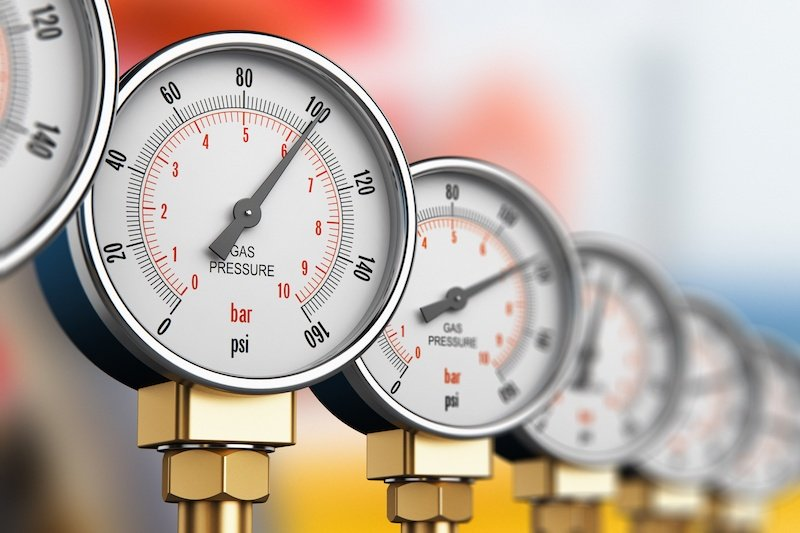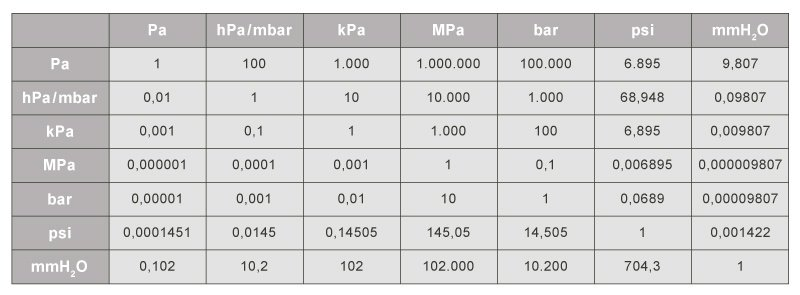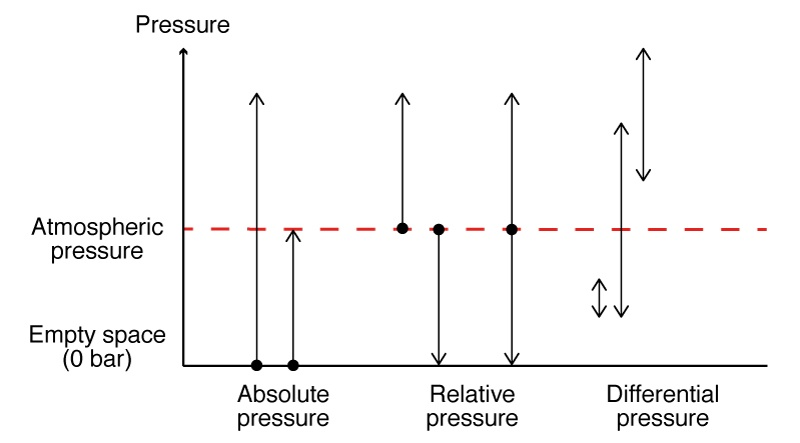# The physical values of pressure and the various forms of pressureBy STS/PMC June 30, 2020

Besides temperature, pressure is one of the most frequently measured physical variables in industrial applications. There exist, however, different measurement units for, and different forms of, the pressure itself. In the following we will explain the basic prevailing terms.

Pressure describes the force (F) acting on a surface (A) and is represented by the formula symbol p:

p = F/A

According to the international system of units, the SI unit of pressure is named the Pascal (Pa). The term stems from the French mathematician Blaise Pascal (1623-1662) and is derived as follows from the SI units of meters and Newtons: 1 Pa = 1 N/m2.

The Pascal is thus a very small unit of pressure. In industrial applications, the unit of bar is therefore generally applied. The units used to indicate pressure also vary from one application area to the next. The Pa is applied for pressure measurements in clean rooms, whereas meteorology relies upon the hPa. Blood pressure, on the other hand, is measured in units of mmHg. How these individual units relate to one other is clearly shown in the conversion chart below.Figure 1: Pressure units conversion chart

The pressure forms

For users, it is important to be able to differentiate the different forms of pressures in order to select the ideal pressure transmitter for their application.

The subdivision into absolute, differential and relative pressure is therefore decisive to pressure measurement.

Absolute pressure

Absolute pressure is referenced to a pressure of zero. This implies an airless space, as exists, for example, in the vastness of the universe or in an ideal vacuum. The measured pressure is therefore always greater than the reference pressure. For a better differentiation from the other forms of pressure, the absolute pressure is indicated with the abs index: Pabs.

Absolute pressure sensors use a vacuum enclosed within the sensor element as a reference pressure. This is situated on the secondary side of the membrane. Besides meteorological applications, absolute pressure sensors are also often used in the packaging industry (e.g., in the manufacture of vacuum packaging).Figures 2: Summary of various pressure forms

Relative pressure (Gage pressure)

Relative pressure is referenced to the atmospheric pressure, which is indicated by the amb index. This is the pressure that acts through the earth-enveloping layer of air. This pressure decreases continuously up to an altitude of about 500 kilometers (from this elevation absolute pressure then prevails). The atmospheric pressure at sea level corresponds to about 1013 mbar and fluctuates by about five percent with high and low pressure conditions.

In contrast to an absolute pressure sensor, the secondary side of a relative pressure sensor remains open, in order to ensure pressure equalization with the atmospheric pressure. In addition to relative pressure, the term overpressure is also common. A positive overpressure is thus referred to when the absolute pressure is higher than the atmospheric pressure. When this is not the case, then a negative overpressure is expressed (previously, the term vacuum was also used).

One practical example of a relative pressure measurement is the tire pressure of a vehicle. If 2 bar of relative pressure is supplied to a tire at an air pressure of 1 bar, this would then correspond to 3 bar in absolute pressure.

Differential pressure

With differential pressure, the difference in pressure between any two pressures is indicated. For this reason, differential pressure sensors have two pressure connections.

One application example for differential pressure measurement is the hydrostatic pressure measurement within enclosed tanks. You can read more on this here.